# Second Order Homogeneous DE

A linear second order homogeneous differential equation involves terms up to the second derivative of a function. For the case of constant multipliers, The equation is of the formand can be solved by the substitution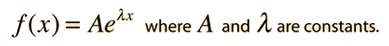The solution which fits a specific physical situation is obtained by substituting the solution into the equation and evaluating the various constants by forcing the solution to fit the physical boundary conditions of the problem at hand. Substituting gives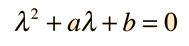which leads to a variety of solutions, depending on the values of a and b. In physical problems, the boundary conditions determine the values of a and b, and the solution to the quadratic equation for λ reveals the nature of the solution.

Case I: Two real roots

For values of a and b such thatthere are two real roots λ1 and λ2 which lead to a general solution of the form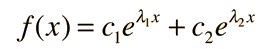Case II: A real double root

If a and b are such thatthen there is a double root λ =-a/2 and the unique form of the general solution is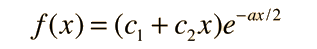Case III: Complex conjugate roots

For values of a and b such thatthere are two complex conjugate roots of the form and the general solution is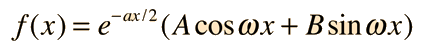The solution to the homogeneous equation is important on its own for many physical applications, and is also a part of the solution of the non-homogeneous equation.

### Applications

Index

References

Kreyzig
Ch 2

 HyperPhysics****HyperMath*****Differential equations R Nave
Go Back

# Differential Equation Applications

These are physical applications of second-order differential equations. There are also many applications of first-order differential equations.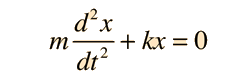Azimuthal equation, hydrogen atom: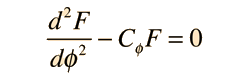Velocity profile in fluid flow.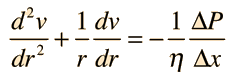Index

References

Kreyzig
Ch 2

 HyperPhysics****HyperMath*****Differential equations R Nave
Go Back

# Applications of 1st Order Homogeneous Differential Equations

The general form of the solution of the homogeneous differential equation can be applied to a large number of physical problems.

 Barometric pressure variation with altitude: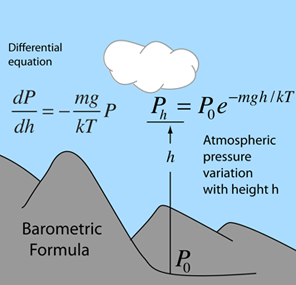Discharge of a capacitor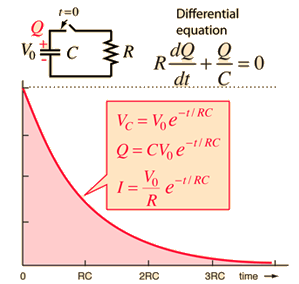Index

References

Kreyzig
Ch 2

 HyperPhysics****HyperMath*****Differential equations R Nave
Go Back